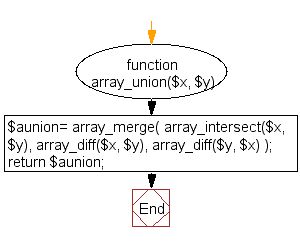﻿ PHP Array Exercise: Set union of two arrays - w3resource# PHP Array Exercises : Set union of two arrays

## PHP Array: Exercise-48 with Solution

Write a PHP function to set union of two arrays.

Sample Solution:

PHP Code:

``````<?php
function array_union(\$x, \$y)
{
\$aunion=  array_merge(
array_intersect(\$x, \$y),
array_diff(\$x, \$y),
array_diff(\$y, \$x)
);
return \$aunion;
}
\$a = array(1, 2, 3, 4);
\$b = array(2, 3, 4, 5, 6);
print_r(array_union(\$a, \$b));
?>
```
```

Sample Output:

```Array
(
 => 2
 => 3
 => 4
 => 1
 => 5
 => 6
)
```

Flowchart:PHP Code Editor:

What is the difficulty level of this exercise?

﻿

## PHP: Tips of the Day

PHP: Extract numbers from a string

```\$str = 'In My Cart : 11 12 items';
preg_match_all('!\d+!', \$str, \$matches);
print_r(\$matches);
```